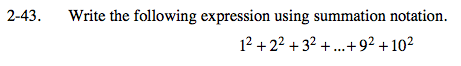### Home > PC > Chapter 2 > Lesson 2.2.1 > Problem2-43

2-43.How many terms are being added? Start your index at 1. Stop at the last term. (Alternatively, you could start at 0 and end at 9.)

$\displaystyle\sum\limits_{k=1}^{10}$

Find the argument that will result in the desired expansion.

$\displaystyle\sum\limits_{k=1}^{10}k^2$